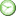# ISEE Math Practice Test: Middle and Upper Levels

### Test Information16 questions0 minutes

Take more free ISEE Mathematics Achievement Practice Tests available from crackssat.com.

1. How many numbers between 1 and 100, inclusive, are both prime and a multiple of 4 ?

• A. 0
• B. 12
• C. 20
• D. 25

2. How many factors do the integers 24 and 81 have in common?

• A. 1
• B. 2
• C. 3
• D. 4

3. If the final total of a dinner bill-after including a 25% tip-is \$50, what was the cost of the dinner before including the tip?

• A. \$12.50
• B. \$25.00
• C. \$37.50
• D. \$40.00

4. How many numbers between 1 and 100 are multiples of both 2 and 7 ?

• A. 6
• B. 7
• C. 8
• D. 9

5. 23 × 23 × 23 =

• A. 26
• B. 29
• C. 227
• D. 89

6. For what integer value of m does 2m + 4 = m3 ?

• A. 1
• B. 2
• C. 3
• D. 4

7. If 6x - 4 = 38, then x + 10 =

• A. 7
• B. 10
• C. 16
• D. 17

8. What is the smallest multiple of 7 that is greater than 50 ?

• A. 7
• B. 49
• C. 51
• D. 56

9. One-fifth of the students in a class chose recycling as the topic for their science projects. If four students chose recycling, how many students are in the class?

• A. 4
• B. 10
• C. 16
• D. 20

10. If a harvest yielded 60 bushels of corn, 20 bushels of wheat, and 40 bushels of soybeans, what percent of the total harvest was corn?

• A. 50%
• B. 40%
• C. 33%
• D. 30%

11. At a local store, an item that usually sells for \$45 is currently on sale for \$30. By what percent is that item discounted?

• A. 10%
• B. 25%
• C. 33%
• D. 50%

12. Which of the following is most nearly 35% of \$19.95 ?

• A. \$3.50
• B. \$5.75
• C. \$7.00
• D. \$9.95

13. A pair of shoes is offered on a special blowout sale. The original price of the shoes is reduced from \$50 to \$20. What is the percent change in the price of the shoes?

• A. 60%
• B. 50%
• C. 40%
• D. 25%

14. Four friends each pay \$5 for a pizza every Friday night. If they were to start inviting a fifth friend to come with them and still bought the same pizza, how much would each person then have to pay?

• A. \$1
• B. \$4
• C. \$5
• D. \$25

15. If the perimeter of a square is 56, what is the length of each side?

• A. 4
• B. 7
• C. 14
• D. 28

16. What is the perimeter of an equilateral triangle, one side of which measures 4 inches?

• A. 12 inches
• B. 8 inches
• C. 6 inches
• D. 4 inches